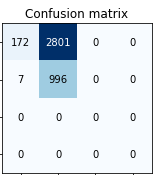# In a multi-label problem CNN Learner is only choosing 2 classes out of 20 classes

Hi, I have a problem with my classifier.

As the title says, my CNN only picks 2 classes out of 20, in my confusion matrix it places 0’s in all other classes. I think the problem is de loss function I have used BCEWithLogitsLossFlat and CrossEntropyLossFlat but with CrossEntropyLossFlat the confusion matrix set 0’s in all the classes, my precision is low and my recall high with both loss functions.

This is the code of my learner
learn = cnn_learner(src, arch = resnet18 ,metrics = [accuracy_multi, PrecisionMulti(average=‘micro’),RecallMulti(average=‘micro’),F1ScoreMulti(average=‘micro’),RocAucMulti(average=‘micro’)] ,normalize=True,pretrained=True, loss_func = BCEWithLogitsLossFlat())

I put a image of the confusion matrix, this is just an example in which I reduced the classes to 4Does anyone know how to solve it or go through the same thing?

Regards

it could be due to the distribution of your data overall or during specific points during the training/validation. Is the model only (or mostly) seeing examples of those two classes during training or is the data being used during validation only of those two classes?

Training set has between 600 and 1500 images each class and the validation set has between 100 a 300 per class. Both sets have images of the 4 classes.

And there are also multi-label images.

Are you having a multilabel or multiclass problem?
multiclass = multiple classes but only one per image
multilabel = mulitple labels for one image
The confusion matrix can only be used, if there is one single label per image. If you have a true multilabel problem, the standard fastai confusion matrix function cannot be applied. However, it will not throw an error but show a matrix similar to yours, which can be confusing.
If you want to use confusion matrices, you need to calculate them per class using a one-against all approach. The `multilabel_confusion_matrix` from scikit-learn does exactly this.

https://scikit-learn.org/stable/modules/generated/sklearn.metrics.multilabel_confusion_matrix.html

2 Likes